# Calculate

## Introduction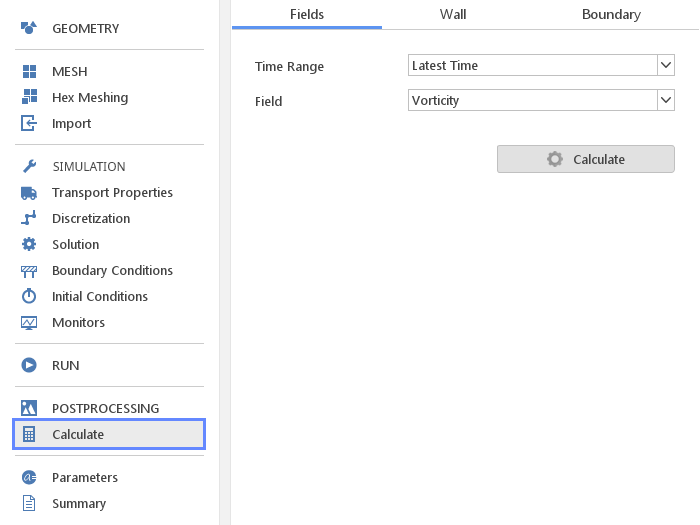This panel allows the basic postprocessing calculations.

Normally output from solvers consists only of the basic fields like pressure, velocity and temperature. It is often necessary to obtain certain derived fields and values. Those additional fields can be obtained in the Calculate panel.

There are three basic types of post-processing calculations:

• Fields - calculates derived fields such as Mach number or vorticity

• Wall - calculates derived fields that are important only on walls, such as $$y^+$$

• Boundary - calculates values at any mesh boundary

All the calculations can be performed on demand using the Calculate button. This button will not be active when there are no results available or the simulation is still running.

## Time Ranges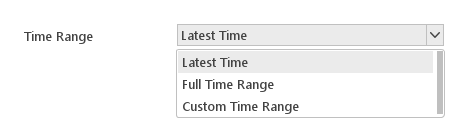By default, calculations are performed only for the latest time. You can change this using the Time Range option.

Options for defining time range:

• Latest Time - performs calculations only for the last time step

• Full Time Range - performs calculations for all available time steps

• Custom Time Range - performs calculations for time steps within the specified range

### Custom Time Ranges Definition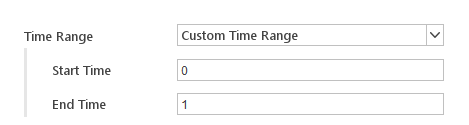When you select Custom Time Range you need to supply two additional values. With these values specified calculations will be performed for time steps between Start Time and End Time .

• Start Time - the lowest time step in the time range

• End Time - the highest time step in the time range

## Calculating Fields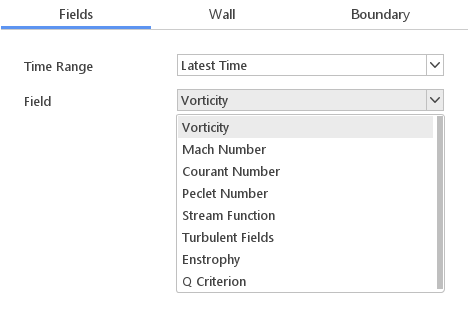In the Fields tab the User is able to calculate additional, following fields:

• Vorticity - vorticity of the velocity field

• Mach Number - Mach number , available only for compressible solvers

• Courant Number - Courant number , meaningful only for transient simulations

• Peclet Number - Peclet number , meaningful only for transient simulations

• Stream Function - stream function , meaningful only for 2D simulations

• Turbulent Fields - calculates missing turbulence fields like $$k$$, $$\epsilon$$, $$\omega$$ and Reynolds stress; available when turbulence model is enabled

• Enstrophy - enstrophy field

• Q Criterion - second invariant of the velocity gradient tensor, $$Q=\frac{{1}}{{2}} [(tr(\nabla U))^2-tr(\nabla U \cdot \nabla U)$$]

## Calculating Wall Values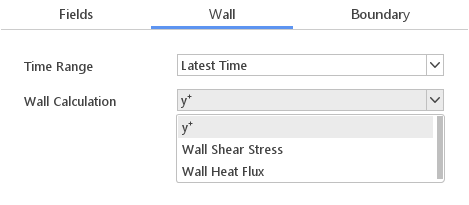The Wall tab calculates the wall related field values.

Wall Calculation Options:

## Calculate Boundary Values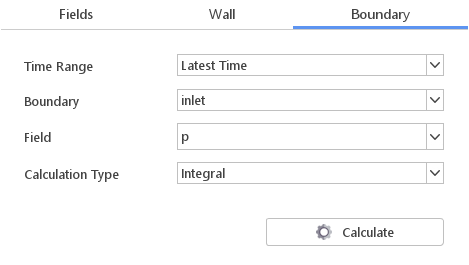The Boundary tab calculates certain integral properties on selected boundaries.

Boundary Calculation Options:

• Boundary - name of the boundary on which the value should be calculated

• Field - name of the field for calculation

• Calculation Type - specifies whether you want to calculate Average or Integral value